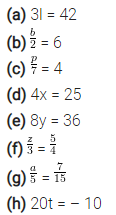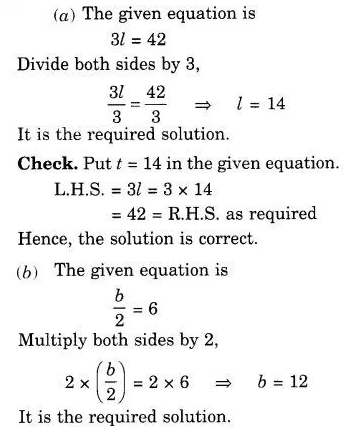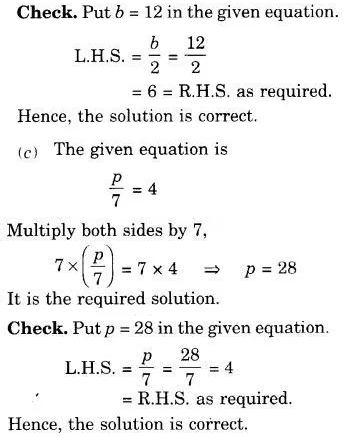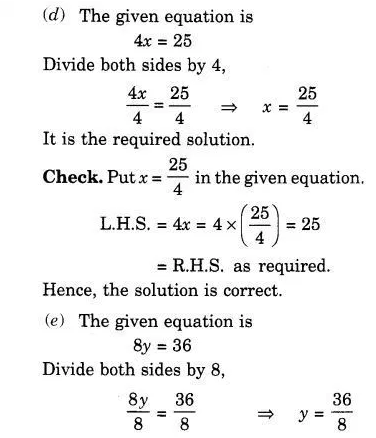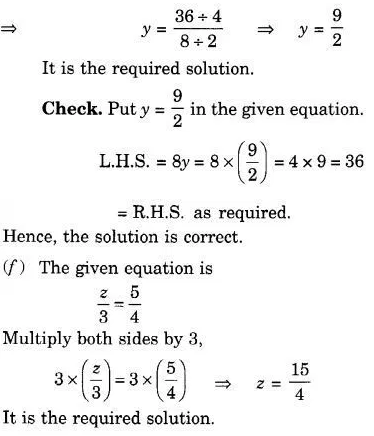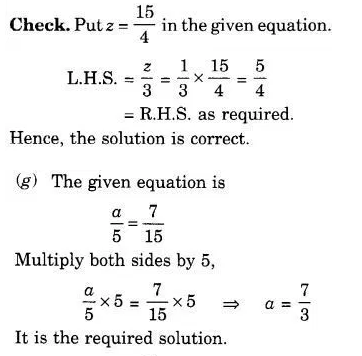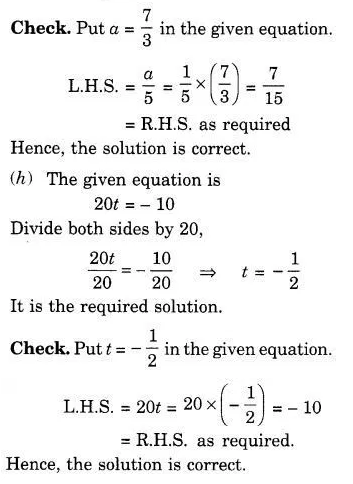# Give first the step you will use to separate the variable and then solve the equation: (a) 3l = 42

Give first the step you will use to separate the variable and then solve the equation: